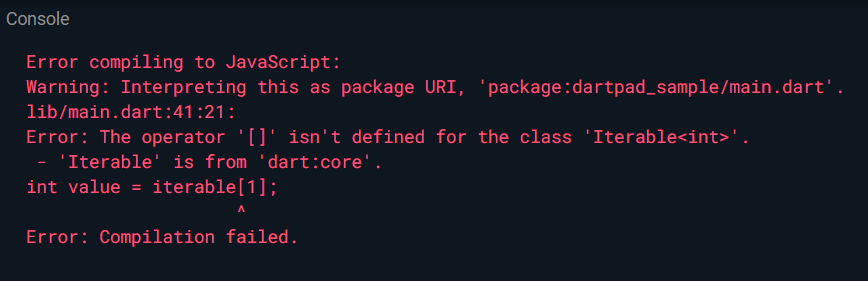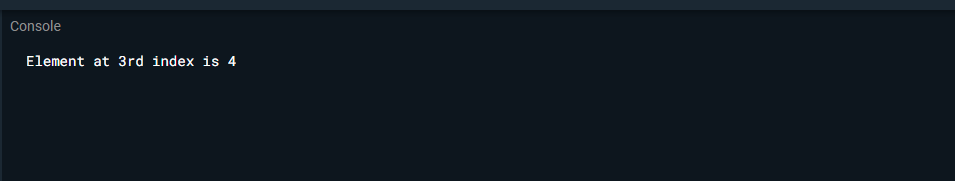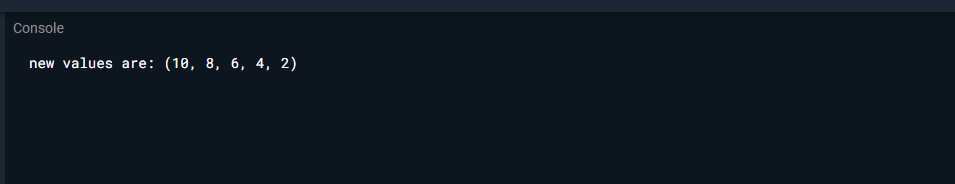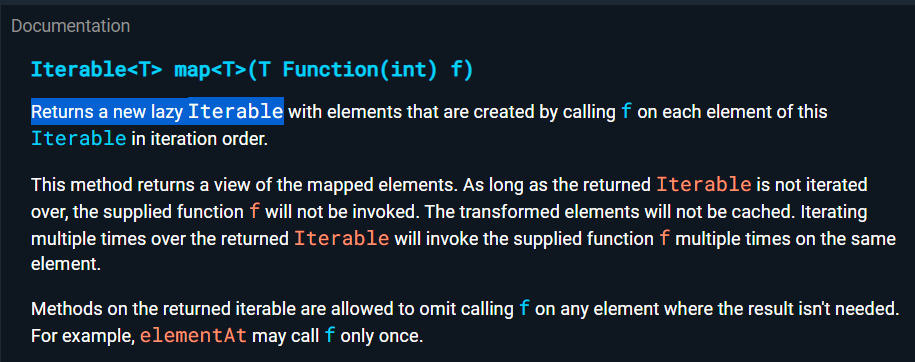# Dart – Advance Concepts of Iterable Collections

• Last Updated : 07 Sep, 2021

In this article, we will look into some of the important concepts related to iterables in Dart.

### 1. Indexing with[ ] operator is invalid:

In dart, there is no operator [ ] in Iterable. To better understand let’s take a look at the below example.

Example:

## Dart

 `void` `main() {``   ` `  ``// invalid program``  ``Iterable<``int``> iterable = [1, 2, 3, 4, 5 ]; ``// line 1``int` `value = iterable; ``// line 2``}`

Output:In this example, we have a list of 5 elements. What if  we want to fetch the number from the particular index?  In line 2 we tried to fetch numbers from the index by writing iterable but the [ ] operator isn’t defined in the class. As you can see the above error we got.

So the question arises how do we fetch the number from a particular index in dart? To do that we use elementAt() in dart.

Syntax:

`elementAt(index_number)`

Here,

• index_number: It is the index whose value is to be fetched.

Take a look at the below example.

Example:

Here we want to fetch the value at index 3. So let’s see how we can do that with the help of elementAt().

## Dart

 `void` `main() {`` ``Iterable<``int``> iterable = [1, 2, 3, 4, 5];`` ``print(``'Element at 3rd index is \${iterable.elementAt(3)}'``);``}`

Output:Explanation: So here we passed index no 3 because we wanted the value stored at that index. So we got the result 4. This is how we can get the value stored at a particular index using elementAt().

### 2. Mapping Iterable

We can map an existing iterable and derive modified values. To do so follow the below steps:

• Take a collection
• Transform all its items according to a condition.
• Return a new collection

Example:

## Dart

 `void` `main() {``  ``const` `list = [5,4,3,2,1];``  ``final value = list.map((number) => number*2);``  ``print(``'new values are: \${value}'``);``}`

Output:### What makes map operators very powerful in dart?

It’s the ability to transform the process into a very short piece of code. Let’s see an example.

Same above code if we write using for loop then we can see below as it takes many lines of code.

## Dart

 `void` `main() {``   ``const` `list = [5,4,3,2,1];``   ` `  ``final element = [];``  ``for``(var value in list){``    ``element.add(value * 2);``  ``}``  ``print(``'new values are: \${element}'``);``}`

Output :

`new values are: [10, 8, 6, 4, 2]`

We see that although the output is the same, the lines of code are more. That’s why a map is powerful because using it we can write code in fewer lines and get the desired result.

### Important note:

According to the official docs, the map returns lazy Iterable.### What does lazy Iterable mean?

Let’s take a look at the below code:

## Dart

 `void` `main() {`` ` ` ``const` `list = [5,4,3,2,1];`` ` ` ``final value = list.map((number) => number*2);`` ` ` ``print(``'new values are: \${value}'``);``}`

Here, the anonymous function isn’t evaluated until the result is used.

This means:

• It means map will not evaluate the anonymous function if the result is not getting printed or it’s isn’t in any use anywhere.
• It’s a performance optimization technique ensuring that all the resulting items are only calculated when needed.

My Personal Notes arrow_drop_up